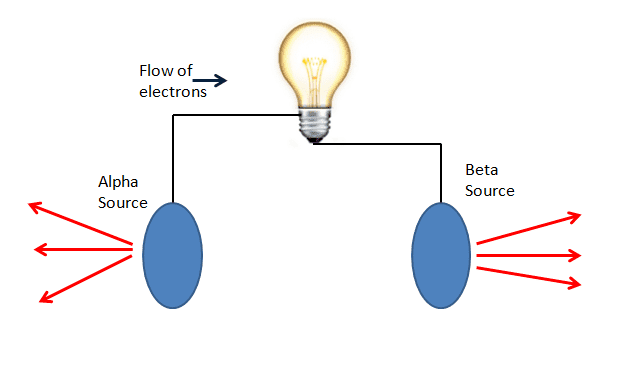# Current Flux from Alpha and Beta Radiation

• B
• Hunter235711
In summary, the smoke detector emits 3.7x10^4 alpha particles per second which carries a current of 11.85fA away from the pellet.f

#### Hunter235711

In a smoke detector there is 1 muCi of Am-241. I'm assuming that means it emits (3.7x10^10)*(1x10^-6)=3.7x10^4 alpha particles per second. Each alpha particle has a charge of 2e, so it seems to me that this stream of particles leaving the Am-241 pellet would carry a current of (3.7x10^4)*2/(6.24x10^18)=11.85fA away from the pellet. Assuming the pellet has a capacitance of 2pF, it would acquire a negative voltage at a rate of 5.9mV/s.

So my question is, if you left such a pellet in a vacuum for an extended period of time would the stream of alpha particles leaving it cause the pellet to charge to a high negative voltage over time?

Thanks,
-Hunter

So my question is, if you left such a pellet in a vacuum for an extended period of time would the stream of alpha particles leaving it cause the pellet to charge to a high negative voltage over time?
In a perfect vacuum: yes. There is no perfect vacuum, however.

Thanks! So in theory at least, you could have a circuit like this?Sure. You don't even need both, just let the radiation of one plate hit the other. This is mainly studied in the context of nuclear fission or fusion, see Direct energy conversion.

if you left such a pellet in a vacuum for an extended period of time would the stream of alpha particles leaving it cause the pellet to charge to a high negative voltage over time?

In a perfect vacuum, as the source becomes more negative, the alphas feel a stronger and stronger force back to the source, and eventually the system reaches a point where the alphas no longer escape. So even in the best possible case, there is a limit.

Alpha particles also ionize electrons from the pellet itself.

Interesting! Thanks for the input. Another question I have is if there is any way to calculate the approximate number of ions formed per second by a radiation source in air? I assume it only depends on the type of radiation, the intensity of radiation, and the pressure of the air.

From this I am interested in calculating the current produced in an ionization chamber for a given voltage across it.

-Hunter